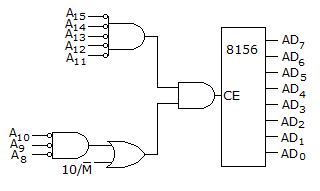# Electronics and Communication Engineering - Digital Electronics

36.

How many zone bits are there?
The 8156 of a figure has RAM locations from 2000 H to 20 FFH.A. 2 B. 4 C. 8 D. 16

Explanation:

No answer description available for this question. Let us discuss.

37.

Which of the following is equivalent to AND-OR realization?

 A. NAND-NOR B. NOR-NOR C. NOR-NAND D. NAND-NAND

Explanation:

No answer description available for this question. Let us discuss.

38.

How many different BCD number can be stored in a register containing 12 switches using an 8, 4, 2, 1 code?

 A. 99 B. 100 C. 999 D. 1000

Explanation:

No answer description available for this question. Let us discuss.

39.

An SR flip flop can be built using

 A. NOR gate only B. NAND gate only C. either NOR or NAND gates D. neither NOR nor NAND gates

Explanation:

No answer description available for this question. Let us discuss.

40.

Which one of the following is correct?

 A. RAM is a volatile memory but ROM is non-volatile memory B. RAM is non-volatile memory but ROM is volatile memory C. Both ROM and RAM are volatile but in ROM data is not lost when power is switched off D. Both ROM and RAM are non-volatile but in RAM data is lost when power is switched off